NEET  >  Test: Introductory Vectors

# Test: Introductory Vectors

Test Description

## 10 Questions MCQ Test Physics Class 11 | Test: Introductory Vectors

Test: Introductory Vectors for NEET 2022 is part of Physics Class 11 preparation. The Test: Introductory Vectors questions and answers have been prepared according to the NEET exam syllabus.The Test: Introductory Vectors MCQs are made for NEET 2022 Exam. Find important definitions, questions, notes, meanings, examples, exercises, MCQs and online tests for Test: Introductory Vectors below.
Solutions of Test: Introductory Vectors questions in English are available as part of our Physics Class 11 for NEET & Test: Introductory Vectors solutions in Hindi for Physics Class 11 course. Download more important topics, notes, lectures and mock test series for NEET Exam by signing up for free. Attempt Test: Introductory Vectors | 10 questions in 10 minutes | Mock test for NEET preparation | Free important questions MCQ to study Physics Class 11 for NEET Exam | Download free PDF with solutions
 1 Crore+ students have signed up on EduRev. Have you?
Test: Introductory Vectors - Question 1

### Find the area of parallelogram formed by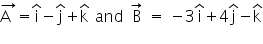Detailed Solution for Test: Introductory Vectors - Question 1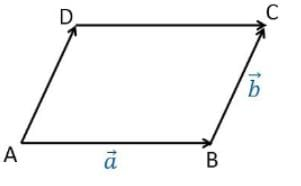Area of Parallelogram = breadth x height⟂ = |(A x B)|

Here, A = i - j + k

And,  B = -3i + 4j -k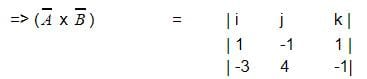= i (1-4) -j (-3+1) +k (4-3)

=> (A x B) = -3i +2j +k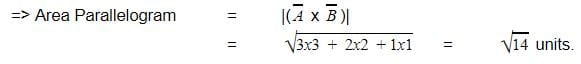Test: Introductory Vectors - Question 2

### A body is projected horizontally from the top of a cliff with a velocity of 9.8m/s. What time elapses before horizontal and vertical velocities become equal? Take g = 9.8m/s2

Detailed Solution for Test: Introductory Vectors - Question 2

Horizontal velocity at any instant, vx = u = 9.8m/s
Vertical velocity at any instant, vy = 0 + gt = 9.8t
9.8 = 9.8t
t = 1s

Test: Introductory Vectors - Question 3

### Two vectors are equal if and only if

Detailed Solution for Test: Introductory Vectors - Question 3

Two vectors are equal if and only if they have the same magnitude and the same direction.
Hence, Option D is correct

Test: Introductory Vectors - Question 4

A cyclist moves along a circular path of radius 70m. If he completes one round in 11s, calculate total length of path.

Detailed Solution for Test: Introductory Vectors - Question 4

Radius of the circular path, r = 70m
Time takes to complete one round, t = 11s
Total length of the path, s = 2πr = 2*22/7*70 = 440m

Test: Introductory Vectors - Question 5

Find the odd one out:

Detailed Solution for Test: Introductory Vectors - Question 5

Force, area and velocity all are a set of different or the same fundamental quantities multiplied or divided together while current itself is a fundamental quantity and can be expressed in terms of any other.

Test: Introductory Vectors - Question 6

An object thrown from an aeroplane is an example for

Detailed Solution for Test: Introductory Vectors - Question 6

A projectile is the name given to any body which once thrown into space with some initial velocity, moves thereafter under the influence of gravity alone without being propelled by an engine or fuel. The path followed by a projectile is called its trajectory.

Test: Introductory Vectors - Question 7

The vector product of parallel vectors is always:

Detailed Solution for Test: Introductory Vectors - Question 7

The vector product of two vectors depends upon the sine of the angle between the vectors and when the vectors are parallel the sine component would be zero hence the resultant is a null vector.

Test: Introductory Vectors - Question 8

Given,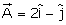and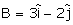. The unit vector of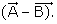is

Detailed Solution for Test: Introductory Vectors - Question 8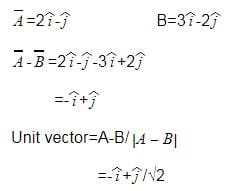Test: Introductory Vectors - Question 9

If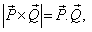what is the angle between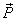and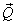Detailed Solution for Test: Introductory Vectors - Question 9

We know that for two vectors P and Q
P.Q = |P||Q| cos a
And PxQ = |P||Q| sin a
Where a is angle between them
When P.Q = PxQ
We get sin a = cos a
Thus a = 450

Test: Introductory Vectors - Question 10

A particle of mass 10 g moves along a circle of radius 6.4 cm with a constant tangential acceleration. What is the magnitude of this acceleration, if the kinetic energy of the particle becomes equal to 8 x 10-4 J by the end of the second revolution after the beginning of the motion?

Detailed Solution for Test: Introductory Vectors - Question 10

kinetic energy =8×10−4J

or, (1/2)​​mv2=8×10−4

or, (1/2)​×10×10−3v²=8×10−4

or, v2=16×10−2

=>v=0.4m/s

initial velocity of particle,   u=0m/s

we have to find Tangential acceleration at the end of 2nd revolution.

total distance covered, s=2(2πr)=4πr

so, v2=2as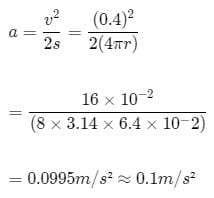## Physics Class 11

126 videos|449 docs|210 tests
 Use Code STAYHOME200 and get INR 200 additional OFF Use Coupon Code
Information about Test: Introductory Vectors Page
In this test you can find the Exam questions for Test: Introductory Vectors solved & explained in the simplest way possible. Besides giving Questions and answers for Test: Introductory Vectors, EduRev gives you an ample number of Online tests for practice

## Physics Class 11

126 videos|449 docs|210 tests

### How to Prepare for NEET

Read our guide to prepare for NEET which is created by Toppers & the best Teachers